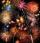# Bricklayers

8 bricklayers build a house for 630 days. How many bricklayers have to add after 150 days, so then the whole building completed in (next) 320 days?

Result

n =  4

#### Solution:

150/630+(n+8)*320/(630*8)=1

40n = 160

n = 4

Calculated by our simple equation calculator.

Leave us a comment of example and its solution (i.e. if it is still somewhat unclear...):

Showing 0 comments:Be the first to comment!#### To solve this example are needed these knowledge from mathematics:

Need help calculate sum, simplify or multiply fractions? Try our fraction calculator. Do you have a linear equation or system of equations and looking for its solution? Or do you have quadratic equation?

## Next similar examples:

1. The bunsKate, Zofia and Peter Liked buns. Even today, their grandmother prepare their favorite meal. Katka eats 4 bunches, Žofia 3 and Petra eats 5 buns. Their grandmother said to them, "My inmate will you know how many buns I have been make today, if those you ea
2. Cleaning windowsCleaning company has to wash all the windows of the school. The first day washes one-sixth of the windows of the school, the next day three more windows than the first day and the remaining 18 windows washes on the third day. Calculate how many windows ha
3. PearsThere were pears in the basket, I took two-fifths of them, and left six in the basket. How many pears did I take?
4. King's birthdayTo celebrate the king's birthday workers fire 1/5 all purchased rockets. To celebrate the Queen's birthday fire 1/6 of the remaining rockets and to celebrate the birthday of king's son remaining 15,000 rockets. How many rockets they purchased?
5. TreesFrom the total number of trees in the orchard, there are two-fifths pearls and apples are three eighty. The rest of the trees are 9 ceremonial. How many trees are in the set?
6. Boys and girlsThere are 28 girls in the hall. 5/7 of all children are boys. How many children and how many boys are there?
7. Unknown numberI think the number. I'll reduce it to its one-third. The result is then increased by one-third, and I get the number 12.
8. Hectoliters of waterThe pool has a total of 126 hectoliters of water. The first pump draws 2.1 liters of water per second. A second pump pumps 3.5 liters of water per second. How long will it take both pumps to drain four-fifths of the water at the same time?
9. Simple equationSolve for x: 3(x + 2) = x - 18
10. Unknown numberI think the number - its sixth is 3 smaller than its third.
11. EqnSolve equation with fractions: 2x/3-50=40+x/4
12. TogetherGrandfather, father, and son are carpenters. They decided to make a wardrobe. Father produces it for 12 hours, his son for 15 hours, and grandfather for 10 hours. How long will they make it together?
13. FractionsThree-quarters of an unknown number are 4/5. What is 5/6 of this unknown number?
14. EquationSolve equation and check the result: 1.4x - 3/2 + x - 9,8 = x + 0,4/3 - 7 + 1,6/6
15. Equation with fractionsSolve equation: ? It is equation with fractions.
16. Unknown number 6Determine the unknown number, which is by 1.5 greater than its fourth.
17. Equation with xSolve the following equation: 2x- (8x + 1) - (x + 2) / 5 = 9